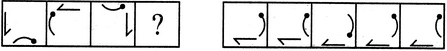# Non Verbal Reasoning - Analogy - Discussion

### Discussion :: Analogy - Section 1 (Q.No.41)

Each of the following questions consists of two sets of figures. Figures A, B, C and D constitute the Problem Set while figures 1, 2, 3, 4 and 5 constitute the Answer Set. There is a definite relationship between figures A and B. Establish a similar relationship between figures C and D by selecting a suitable figure from the Answer Set that would replace the question mark (?) in fig. (D).

41.

Select a suitable figure from the Answer Figures that would replace the question mark (?).(A)     (B)      (C)     (D)                  (1)      (2)      (3)      (4)      (5)

 [A]. 1 [B]. 2 [C]. 3 [D]. 4 [E]. 5

Explanation:

The half-arrow rotates 90oACW and gets laterally inverted and moves to the adjacent side of the square boundary in a CW direction. The bent pin rotates 90oACW and moves to the adjacent side of the square boundary in a CW direction.

 Daljit said: (Jun 6, 2014) What is ACW?

 Souvik said: (Sep 20, 2015) Please tell me in a simple way.

 Anonyms said: (Aug 28, 2016) It is very difficult to analyze the figs, please tell me the simple trick.

 Pujasam said: (Dec 29, 2018) ACW=anticlockwise.

 Aryan Xettri said: (Aug 3, 2021) I think the right answer is 'C'.